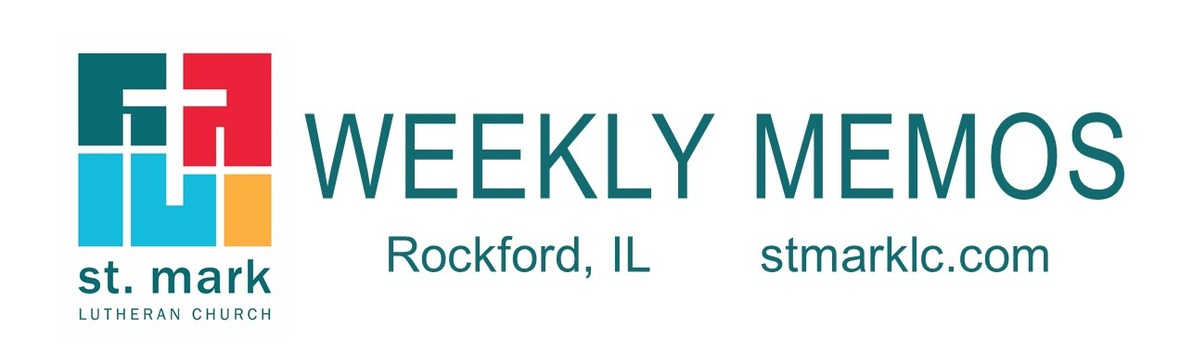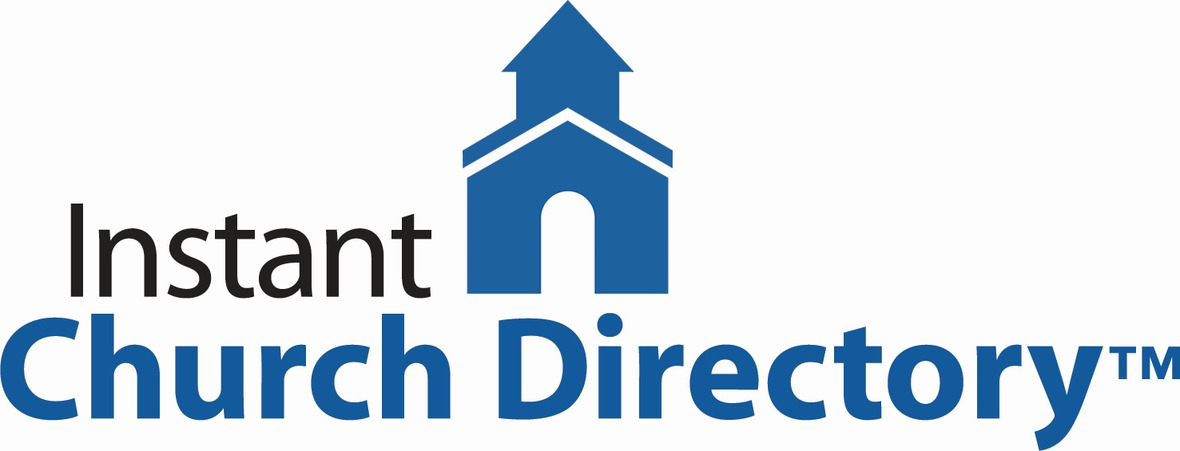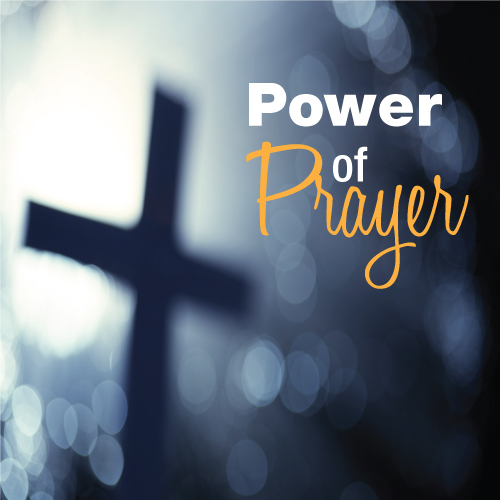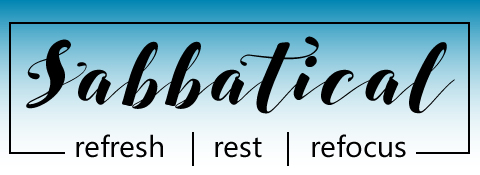Like   Tweet/* styles */
 table div table+table+table div table{width:100%;padding:0}table div table+table+table div table img{width:96.23%;padding:0;float:none}table div table+table+table div table td{width:100%;padding:0 1.88% 18px}/* styles */## Sunday, September 15 Ministry Fair

8:00 A.M. Worship
9:30 A.M. Worship
9:30 A.M. Installation of Sunday School Teachers
9:30 A.M. Sunday School
11:00 A.M. Worship

## Monday, September 16

9:00 A.M. Classic Chorale Rehearses
4:00 P.M. Deadline for MEMOS articles to be submitted
6:30 P.M. Blue Lights
6:30 P.M. Emotions Anonymous
7:00 P.M. Theology Pub

## Tuesday, September 17

10:00 A.M. Staff Meeting
11:00 A.M. St. Mark Duplicate Bridge
6:30 P.M. Executive Meeting

## Wednesday, September 18

9:00 A.M. Women's Bible Study
9:00 A.M. Men's Bible Study
9:30 A.M. St. Mark Ringers
5:30 P.M. Good News Bearers
6:00 P.M. Bible Study
6:30 P.M. Confirmation
7:00 P.M. Voices of St. Mark

## Thursday, September 19

9:00 A.M. Cherry Valley Bridge Group
2:00 P.M. Barnabas Meeting
6:00 P.M. Diakonia
6:30 P.M. Emotions Anonymous
7:00 P.M. Praise Band Rehearses

## Friday, September 20

7:30 A.M. St. Mark Men's Breakfast at Denny's
9:00 A.M. Visitation for Naomi Hagen
11:00 A.M. Memorial Service for Naomi Hagen
12:00 P.M. Luncheon / Fellowship Hour

## Sunday, September 22

8:00 A.M. Worship
9:30 A.M. Worship
9:30 A.M. Sunday School
11:00 A.M. Worship

 table div table+table+table+table+table+table+table div table{width:100%;padding:0}table div table+table+table+table+table+table+table div table img{width:96.23%;padding:0;float:none}table div table+table+table+table+table+table+table div table td{width:100%;padding:0 1.88% 18px}/* styles */## News and Events

 table div table+table+table+table+table+table+table+table+table div table{width:100%;padding:0}table div table+table+table+table+table+table+table+table+table div table img{width:96.23%;padding:0;float:none}table div table+table+table+table+table+table+table+table+table div table td{width:100%;padding:0 1.88% 18px}/* styles *//* styles */ Have you been praying about finding your place at St. Mark, a place to share your talents or learn new ones or even discover new opportunities? We encourage you to stop in Fellowship Hall between services on Sunday, September 15 and see as many tables as you can. There will be information for you to take with you, sign-up sheets and smiling faces. Get involved and recommit to your ministry or committee. You won't be disappointed.
 table div table+table+table+table+table+table+table+table+table+table+table+table div table{width:100%;padding:0}table div table+table+table+table+table+table+table+table+table+table+table+table div table img{width:96.23%;padding:0;float:none}table div table+table+table+table+table+table+table+table+table+table+table+table div table td{width:100%;padding:0 1.88% 18px}/* styles */Our online church directory is looking better and better every day. If you have not submitted your family photo please do so by following the link below. Also, you can download the Instant Church Directory app now.

Instant Church Directory

Once you have downloaded the app and created an account, you are not able to change or update your information, the system administrator is the only one to change and update the information. If something is incorrect, please contact the church office at 815-398-3557 or email jill@stmarklc.com.

Instant Church Directory is a simple way to view your Church Directory. Never deal with an outdated directory again, any changes made by church staff are immediately synced to the app.

Mobile App Login Instructions for Android & Kindle Devices

We also have a form for you to complete to make sure we have the most current information for the directory. There are paper forms at church or click on the link to Church Directory Form

 table div table+table+table+table+table+table+table+table+table+table+table+table+table+table div table{width:100%;padding:0}table div table+table+table+table+table+table+table+table+table+table+table+table+table+table div table img{width:96.23%;padding:0;float:none}table div table+table+table+table+table+table+table+table+table+table+table+table+table+table div table td{width:100%;padding:0 1.88% 18px}/* styles */Some of you have expressed concern about not having access to the new online church directory and wondering if we could print one for you. The answer is yes. We will be printing a few directories for those who have no access online or have an email account. We will let you know when this will be.

 table div table+table+table+table+table+table+table+table+table+table+table+table+table+table+table+table div table{width:100%;padding:0}table div table+table+table+table+table+table+table+table+table+table+table+table+table+table+table+table div table img{width:96.23%;padding:0;float:none}table div table+table+table+table+table+table+table+table+table+table+table+table+table+table+table+table div table td{width:100%;padding:0 1.88% 18px}/* styles */Calling All Musicians!
Bob Rub would like to put together an instrumental ensemble to play and accompany some pieces at St. Mark’s Christmas concert on December 8, 2019. The concert is at 3:00 p.m. If you play an instrument and would like to be a part of the concert, please email Bob at bob@stmarklc.com. In general, High school-aged youth through adults are encouraged to participate. Younger students who play and read music well will also be welcome. There will be a few rehearsals, but we can work out scheduling when we know who is available. Thank you for your consideration.

 table div table+table+table+table+table+table+table+table+table+table+table+table+table+table+table+table+table+table div table{width:100%;padding:0}table div table+table+table+table+table+table+table+table+table+table+table+table+table+table+table+table+table+table div table img{width:96.23%;padding:0;float:none}table div table+table+table+table+table+table+table+table+table+table+table+table+table+table+table+table+table+table div table td{width:100%;padding:0 1.88% 18px}/* styles */Theology Pub
Join Pastor Chad for a pint and great conversation at Carlyle Brewing Co. (215 East State St.) on Monday, September 16 at 7:00 P.M.

 table div table+table+table+table+table+table+table+table+table+table+table+table+table+table+table+table+table+table+table+table div table{width:100%;padding:0}table div table+table+table+table+table+table+table+table+table+table+table+table+table+table+table+table+table+table+table+table div table img{width:96.23%;padding:0;float:none}table div table+table+table+table+table+table+table+table+table+table+table+table+table+table+table+table+table+table+table+table div table td{width:100%;padding:0 1.88% 18px}/* styles */Panera Book Study Beginning October 10
Join Pastor Mark as he begins Panera Book Study on October 10. They meet at Panera at 609 S. Perryville, Rockford at 7:30 A.M. on Thursdays. The book will be Incarnation by William H. Willimon.

 table div table+table+table+table+table+table+table+table+table+table+table+table+table+table+table+table+table+table+table+table+table+table div table{width:100%;padding:0}table div table+table+table+table+table+table+table+table+table+table+table+table+table+table+table+table+table+table+table+table+table+table div table img{width:96.23%;padding:0;float:none}table div table+table+table+table+table+table+table+table+table+table+table+table+table+table+table+table+table+table+table+table+table+table div table td{width:100%;padding:0 1.88% 18px}/* styles */St. Mark Prayer Chain
Would you like to be a part of the St. Mark prayer chain? Prayer requests come into the church office weekly. As we receive prayer requests we would send out an e-mail and ask that you pray daily for the persons mentioned in the requests. All requests are to remain confidential. Please e-mail melinda@stmarklc.com if you would like to participate in the prayer chain. “Be anxious for nothing, but in everything by prayer and supplication, with thanksgiving, let your requests be made known to God.” Colossians 4:2.

 table div table+table+table+table+table+table+table+table+table+table+table+table+table+table+table+table+table+table+table+table+table+table+table+table div table{width:100%;padding:0}table div table+table+table+table+table+table+table+table+table+table+table+table+table+table+table+table+table+table+table+table+table+table+table+table div table img{width:96.23%;padding:0;float:none}table div table+table+table+table+table+table+table+table+table+table+table+table+table+table+table+table+table+table+table+table+table+table+table+table div table td{width:100%;padding:0 1.88% 18px}/* styles */Northern Illinois Food Bank “Packing” Day.
Join us Friday, September 27. There is a morning shift 9:30-11: 30 A.M. or a 3:00-5: 00 P.M. To register for this event go on the Northern Illinois Food Bank site.

 table div table+table+table+table+table+table+table+table+table+table+table+table+table+table+table+table+table+table+table+table+table+table+table+table+table+table div table{width:100%;padding:0}table div table+table+table+table+table+table+table+table+table+table+table+table+table+table+table+table+table+table+table+table+table+table+table+table+table+table div table img{width:96.23%;padding:0;float:none}table div table+table+table+table+table+table+table+table+table+table+table+table+table+table+table+table+table+table+table+table+table+table+table+table+table+table div table td{width:100%;padding:0 1.88% 18px}/* styles */table div table+table+table+table+table+table+table+table+table+table+table+table+table+table+table+table+table+table+table+table+table+table+table+table+table+table+table div table,table.module-26{width:3.77%;float:left;padding:0}table div table+table+table+table+table+table+table+table+table+table+table+table+table+table+table+table+table+table+table+table+table+table+table+table+table+table+table div table a{border:0 none;text-decoration:none}table div table+table+table+table+table+table+table+table+table+table+table+table+table+table+table+table+table+table+table+table+table+table+table+table+table+table+table div table img{width:100%!important;border:0 none;text-decoration:none}table div table+table+table+table+table+table+table+table+table+table+table+table+table+table+table+table+table+table+table+table+table+table+table+table+table+table+table div table td{width:100%;padding:0 20px 20px 0}/* styles */ Are you set up for automatic giving? We are in the process of changing our giving platform. Watch your emails in the next few weeks regarding the change. We will have plenty of instructions on how this will take place and when.
 table div table+table+table+table+table+table+table+table+table+table+table+table+table+table+table+table+table+table+table+table+table+table+table+table+table+table+table+table div table{width:100%;padding:0}table div table+table+table+table+table+table+table+table+table+table+table+table+table+table+table+table+table+table+table+table+table+table+table+table+table+table+table+table div table img{width:96.23%;padding:0;float:none}table div table+table+table+table+table+table+table+table+table+table+table+table+table+table+table+table+table+table+table+table+table+table+table+table+table+table+table+table div table td{width:100%;padding:0 1.88% 18px}/* styles */God’s work. Our hands. Sock and underwear drive for “Just Breathe 815.” Donations are accepted September 1-15th. Adult size socks and underwear for young adults are needed. See the collection bin in the Fellowship Hall. Just Breathe 815 is a resource center to foster social, academic, and independent healthy life-styles for homeless teens.

Do you have a youth who needs/wants service hours? We have two upcoming opportunities for volunteering on Sunday, September 8, and Sunday, September 15th. Please see Melinda Alekna or e-mail melinda@stmarklc.com

 table div table+table+table+table+table+table+table+table+table+table+table+table+table+table+table+table+table+table+table+table+table+table+table+table+table+table+table+table+table+table div table{width:100%;padding:0}table div table+table+table+table+table+table+table+table+table+table+table+table+table+table+table+table+table+table+table+table+table+table+table+table+table+table+table+table+table+table div table img{width:96.23%;padding:0;float:none}table div table+table+table+table+table+table+table+table+table+table+table+table+table+table+table+table+table+table+table+table+table+table+table+table+table+table+table+table+table+table div table td{width:100%;padding:0 1.88% 18px}/* styles */Parents of college students - do you have a young person that is going to college or in the military? Do you think they would enjoy receiving a “CARE?”
Please send in their name, address, and the college they are attending or military address. E-mail melinda@stmarklc.com by October 15. We would love to send them some treats, some notes of encouragement and well wishes from St. Mark.

 table div table+table+table+table+table+table+table+table+table+table+table+table+table+table+table+table+table+table+table+table+table+table+table+table+table+table+table+table+table+table+table+table div table{width:100%;padding:0}table div table+table+table+table+table+table+table+table+table+table+table+table+table+table+table+table+table+table+table+table+table+table+table+table+table+table+table+table+table+table+table+table div table img{width:96.23%;padding:0;float:none}table div table+table+table+table+table+table+table+table+table+table+table+table+table+table+table+table+table+table+table+table+table+table+table+table+table+table+table+table+table+table+table+table div table td{width:100%;padding:0 1.88% 18px}/* styles */Day trip to Geneva, Illinois (near St. Charles) Invite your friends, neighbor, co-workers to join us Saturday, November 9. We will travel by coach bus and the cost is \$35. Sign-up starts this Sunday at our ministry fair. Geneva has beautiful shops, restaurants, art galleries, chocolate shops. Watch for more details as they become available.

 table div table+table+table+table+table+table+table+table+table+table+table+table+table+table+table+table+table+table+table+table+table+table+table+table+table+table+table+table+table+table+table+table+table+table div table{width:100%;padding:0}table div table+table+table+table+table+table+table+table+table+table+table+table+table+table+table+table+table+table+table+table+table+table+table+table+table+table+table+table+table+table+table+table+table+table div table img{width:96.23%;padding:0;float:none}table div table+table+table+table+table+table+table+table+table+table+table+table+table+table+table+table+table+table+table+table+table+table+table+table+table+table+table+table+table+table+table+table+table+table div table td{width:100%;padding:0 1.88% 18px}/* styles */The Good News Bearers Return!
Does your child love to perform? Our drama ministry for students in 2nd-6th grade begins again on Wednesday, September 18th, 5:30-6: 30 pm. We meet most every Wednesday and perform at the 9:30 service about every 6 weeks. Pastor Chad leads the group with theatre games, rehearsals, and lots of fun! If your child would join the Good News Bearers this year, please let Pastor Chad know.

 table div table+table+table+table+table+table+table+table+table+table+table+table+table+table+table+table+table+table+table+table+table+table+table+table+table+table+table+table+table+table+table+table+table+table+table+table div table{width:100%;padding:0}table div table+table+table+table+table+table+table+table+table+table+table+table+table+table+table+table+table+table+table+table+table+table+table+table+table+table+table+table+table+table+table+table+table+table+table+table div table img{width:96.23%;padding:0;float:none}table div table+table+table+table+table+table+table+table+table+table+table+table+table+table+table+table+table+table+table+table+table+table+table+table+table+table+table+table+table+table+table+table+table+table+table+table div table td{width:100%;padding:0 1.88% 18px}/* styles */Wednesday Morning Women's and Men's Bible Study
The Wednesday Morning Women’s Bible Study will resume on September 18th. We meet in the conference room in the Conference room from 9:00 – 10:00 A.M. This fall we will begin our year with a study on Angels: Who They Are and How They Help! We will follow that with an overview of Mormonism- their history, practices, and beliefs. All are welcome to join us. Our Bible study is based on Scripture, discussion, and fellowship! If you have any questions please call Deb Iverson at 815-398-9812.

The Wednesday Morning Men's Bible Study will resume on September 18. We will meet in the Common Grounds Cafe from 9:00 - 10:00 A.M. All are welcome to join us. Contact Pastor Chad McKenna for more information at 815-398-3557 or prmckenna@stmarklc.com.

 table div table+table+table+table+table+table+table+table+table+table+table+table+table+table+table+table+table+table+table+table+table+table+table+table+table+table+table+table+table+table+table+table+table+table+table+table+table+table div table{width:100%;padding:0}table div table+table+table+table+table+table+table+table+table+table+table+table+table+table+table+table+table+table+table+table+table+table+table+table+table+table+table+table+table+table+table+table+table+table+table+table+table+table div table img{width:96.23%;padding:0;float:none}table div table+table+table+table+table+table+table+table+table+table+table+table+table+table+table+table+table+table+table+table+table+table+table+table+table+table+table+table+table+table+table+table+table+table+table+table+table+table div table td{width:100%;padding:0 1.88% 18px}/* styles */Margaret Dean will be playing an organ concert at Our Savior’s Lutheran Church in Rockford on Sunday, September 22 at 7:00 p.m. The event is part of Our Savior’s concert series. Please come out to show your support for Margaret and experience a wonderful evening of pipe organ music.

 table div table+table+table+table+table+table+table+table+table+table+table+table+table+table+table+table+table+table+table+table+table+table+table+table+table+table+table+table+table+table+table+table+table+table+table+table+table+table+table+table div table{width:100%;padding:0}table div table+table+table+table+table+table+table+table+table+table+table+table+table+table+table+table+table+table+table+table+table+table+table+table+table+table+table+table+table+table+table+table+table+table+table+table+table+table+table+table div table img{width:96.23%;padding:0;float:none}table div table+table+table+table+table+table+table+table+table+table+table+table+table+table+table+table+table+table+table+table+table+table+table+table+table+table+table+table+table+table+table+table+table+table+table+table+table+table+table+table div table td{width:100%;padding:0 1.88% 18px}/* styles */Pastor Mark Hagen's Sabbatical began on July 1, and he plans to be back in the church office on September 25. Since he will be unavailable during this time, please contact Pastor Chad and the church office for any pastoral needs.

 table div table+table+table+table+table+table+table+table+table+table+table+table+table+table+table+table+table+table+table+table+table+table+table+table+table+table+table+table+table+table+table+table+table+table+table+table+table+table+table+table+table+table div table{width:100%;padding:0}table div table+table+table+table+table+table+table+table+table+table+table+table+table+table+table+table+table+table+table+table+table+table+table+table+table+table+table+table+table+table+table+table+table+table+table+table+table+table+table+table+table+table div table img{width:96.23%;padding:0;float:none}table div table+table+table+table+table+table+table+table+table+table+table+table+table+table+table+table+table+table+table+table+table+table+table+table+table+table+table+table+table+table+table+table+table+table+table+table+table+table+table+table+table+table div table td{width:100%;padding:0 1.88% 18px}/* styles */Yoga can help build flexibility, strength, endurance, and coordination, as well as relieve stress and anxiety, and improve mindfulness. Using breath and movement, this flowing style of yoga is for both beginners and those with some yoga experience. Watch for more information!

 table div table+table+table+table+table+table+table+table+table+table+table+table+table+table+table+table+table+table+table+table+table+table+table+table+table+table+table+table+table+table+table+table+table+table+table+table+table+table+table+table+table+table+table+table div table{width:100%;padding:0}table div table+table+table+table+table+table+table+table+table+table+table+table+table+table+table+table+table+table+table+table+table+table+table+table+table+table+table+table+table+table+table+table+table+table+table+table+table+table+table+table+table+table+table+table div table img{width:96.23%;padding:0;float:none}table div table+table+table+table+table+table+table+table+table+table+table+table+table+table+table+table+table+table+table+table+table+table+table+table+table+table+table+table+table+table+table+table+table+table+table+table+table+table+table+table+table+table+table+table div table td{width:100%;padding:0 1.88% 18px}/* styles */## Children's MinistrySunday SchooBegins Sunday, September 15l!
We welcome all students to Sunday School, ages 3 through 6th grade. If you have not registered your student, please stop by the table in Fellowship Hall or you can register online. www.stmarklc.com/ministries. There is no fee for Sunday School, just an information sheet that we need for each child.

We are so excited to begin a new program this year, learning about God’s love and sharing it with the world!

## Youth Ministry

 table div table+table+table+table+table+table+table+table+table+table+table+table+table+table+table+table+table+table+table+table+table+table+table+table+table+table+table+table+table+table+table+table+table+table+table+table+table+table+table+table+table+table+table+table+table+table+table+table div table,table.module-47{width:3.77%;float:right;padding:0}table div table+table+table+table+table+table+table+table+table+table+table+table+table+table+table+table+table+table+table+table+table+table+table+table+table+table+table+table+table+table+table+table+table+table+table+table+table+table+table+table+table+table+table+table+table+table+table+table div table a{border:0 none;text-decoration:none}table div table+table+table+table+table+table+table+table+table+table+table+table+table+table+table+table+table+table+table+table+table+table+table+table+table+table+table+table+table+table+table+table+table+table+table+table+table+table+table+table+table+table+table+table+table+table+table+table div table img{width:100%!important;border:0 none;text-decoration:none}table div table+table+table+table+table+table+table+table+table+table+table+table+table+table+table+table+table+table+table+table+table+table+table+table+table+table+table+table+table+table+table+table+table+table+table+table+table+table+table+table+table+table+table+table+table+table+table+table div table td{width:100%;padding:0 0 20px 20px}/* styles */ Second Sunday has kicked off and is looking forward to Sunday, October 13, 7:00 P.M. at Katie’s Cup. This event is for all high school youth. Come join us at Second Sunday! There will be time to visit with friends, games, and youth-led worship. Need a ride or more information? See Melinda Alekna 815-871-0390.
 table div table+table+table+table+table+table+table+table+table+table+table+table+table+table+table+table+table+table+table+table+table+table+table+table+table+table+table+table+table+table+table+table+table+table+table+table+table+table+table+table+table+table+table+table+table+table+table+table+table div table{width:100%;padding:0}table div table+table+table+table+table+table+table+table+table+table+table+table+table+table+table+table+table+table+table+table+table+table+table+table+table+table+table+table+table+table+table+table+table+table+table+table+table+table+table+table+table+table+table+table+table+table+table+table+table div table img{width:96.23%;padding:0;float:none}table div table+table+table+table+table+table+table+table+table+table+table+table+table+table+table+table+table+table+table+table+table+table+table+table+table+table+table+table+table+table+table+table+table+table+table+table+table+table+table+table+table+table+table+table+table+table+table+table+table div table td{width:100%;padding:0 1.88% 18px}/* styles */## Barnabas Ministry

 table div table+table+table+table+table+table+table+table+table+table+table+table+table+table+table+table+table+table+table+table+table+table+table+table+table+table+table+table+table+table+table+table+table+table+table+table+table+table+table+table+table+table+table+table+table+table+table+table+table+table+table div table{width:100%;padding:0}table div table+table+table+table+table+table+table+table+table+table+table+table+table+table+table+table+table+table+table+table+table+table+table+table+table+table+table+table+table+table+table+table+table+table+table+table+table+table+table+table+table+table+table+table+table+table+table+table+table+table+table div table img{width:96.23%;padding:0;float:none}table div table+table+table+table+table+table+table+table+table+table+table+table+table+table+table+table+table+table+table+table+table+table+table+table+table+table+table+table+table+table+table+table+table+table+table+table+table+table+table+table+table+table+table+table+table+table+table+table+table+table+table div table td{width:100%;padding:0 1.88% 18px}/* styles *//* styles */ Dear Friend – I was thinking…an elder named Bob said to me…about when I was a boy in the Depression. The only way we could have milk was to steal it. So when the milk truck came into the neighborhood we would jump in, steal some milk, and jump off. Know what I just realized? Every time the driver saw us he’d slow down for us. He let us do that. How many times was exactly the right person there for you? NOW, could you be that person for an elder at St. Mark? So many have no one and visiting just once a month could mean the world to them. You could be the ONLY visitor that month. Let me introduce you to an elder, enjoy, and meet – someone who needs you now. May the Lord watch…Pastor Chuck Please come to our next Reunion Group on Thursday, September 19th, 2:00 in the café! And call me at 815-342-6064 for more info!
 table div table+table+table+table+table+table+table+table+table+table+table+table+table+table+table+table+table+table+table+table+table+table+table+table+table+table+table+table+table+table+table+table+table+table+table+table+table+table+table+table+table+table+table+table+table+table+table+table+table+table+table+table+table div table{width:100%;padding:0}table div table+table+table+table+table+table+table+table+table+table+table+table+table+table+table+table+table+table+table+table+table+table+table+table+table+table+table+table+table+table+table+table+table+table+table+table+table+table+table+table+table+table+table+table+table+table+table+table+table+table+table+table+table div table img{width:96.23%;padding:0;float:none}table div table+table+table+table+table+table+table+table+table+table+table+table+table+table+table+table+table+table+table+table+table+table+table+table+table+table+table+table+table+table+table+table+table+table+table+table+table+table+table+table+table+table+table+table+table+table+table+table+table+table+table+table+table div table td{width:100%;padding:0 1.88% 18px}/* styles */## Our St. Mark members:

Jim Tooley, Jerry Cazaniga, David Young, Keith Haggestad.

## Our condolences to:

Pastor Mark Hagen, Brett and David upon the death of his wife and their mother, Naomi.

## Our condolences to:

Mark and Kristin Hatch and family upon the death of Mark's sister, Sheila Kane.

## Family and friends of St. Mark members:

Eva Love Sherbondy, Matthew and David Lundine, Betty Anderson, Jennifer Peterson, Doug Nelson, Lynn Reed, Janelle Taylor, Dale Tallmadge, Katelyn Audette, Pastor Jane McChesney, Earl Karau, Ann Eck, Clay Heinrich, Denise Nichols, Mary Graham, Barbara Heckart, Kim Menke, Norma Comstock, Scott Jones, Ethan Whitney, Carolyn King, Tom Severson, Katie Butera, Ashton Henley, Nathan Gyllin.

## Those serving in the military and their families:

Tristan Davenport, Scot Hornick, Bailey Panjkovich, Xander Gehrke, Jim Tammen, Brandon Ballenger, Taylor Eversole, Dean Barron.

 table div table+table+table+table+table+table+table+table+table+table+table+table+table+table+table+table+table+table+table+table+table+table+table+table+table+table+table+table+table+table+table+table+table+table+table+table+table+table+table+table+table+table+table+table+table+table+table+table+table+table+table+table+table+table+table+table div table{width:100%;padding:0}table div table+table+table+table+table+table+table+table+table+table+table+table+table+table+table+table+table+table+table+table+table+table+table+table+table+table+table+table+table+table+table+table+table+table+table+table+table+table+table+table+table+table+table+table+table+table+table+table+table+table+table+table+table+table+table+table div table img{width:96.23%;padding:0;float:none}table div table+table+table+table+table+table+table+table+table+table+table+table+table+table+table+table+table+table+table+table+table+table+table+table+table+table+table+table+table+table+table+table+table+table+table+table+table+table+table+table+table+table+table+table+table+table+table+table+table+table+table+table+table+table+table+table div table td{width:100%;padding:0 1.88% 18px}/* styles *//* styles */ Office Hours Monday - Friday, 8:00 A.M. - 4:00 P.M. Telephone: 815-398-3557 Publications St. Mark Memos, the weekly e-newsletter, is distributed each Thursday. The deadline for submission is Monday by 4:00 P.M. Please click to send submissions. Sermons Online Miss a Sunday at St. Mark? Please visit our website and watch the sermons online. Please click to watch.